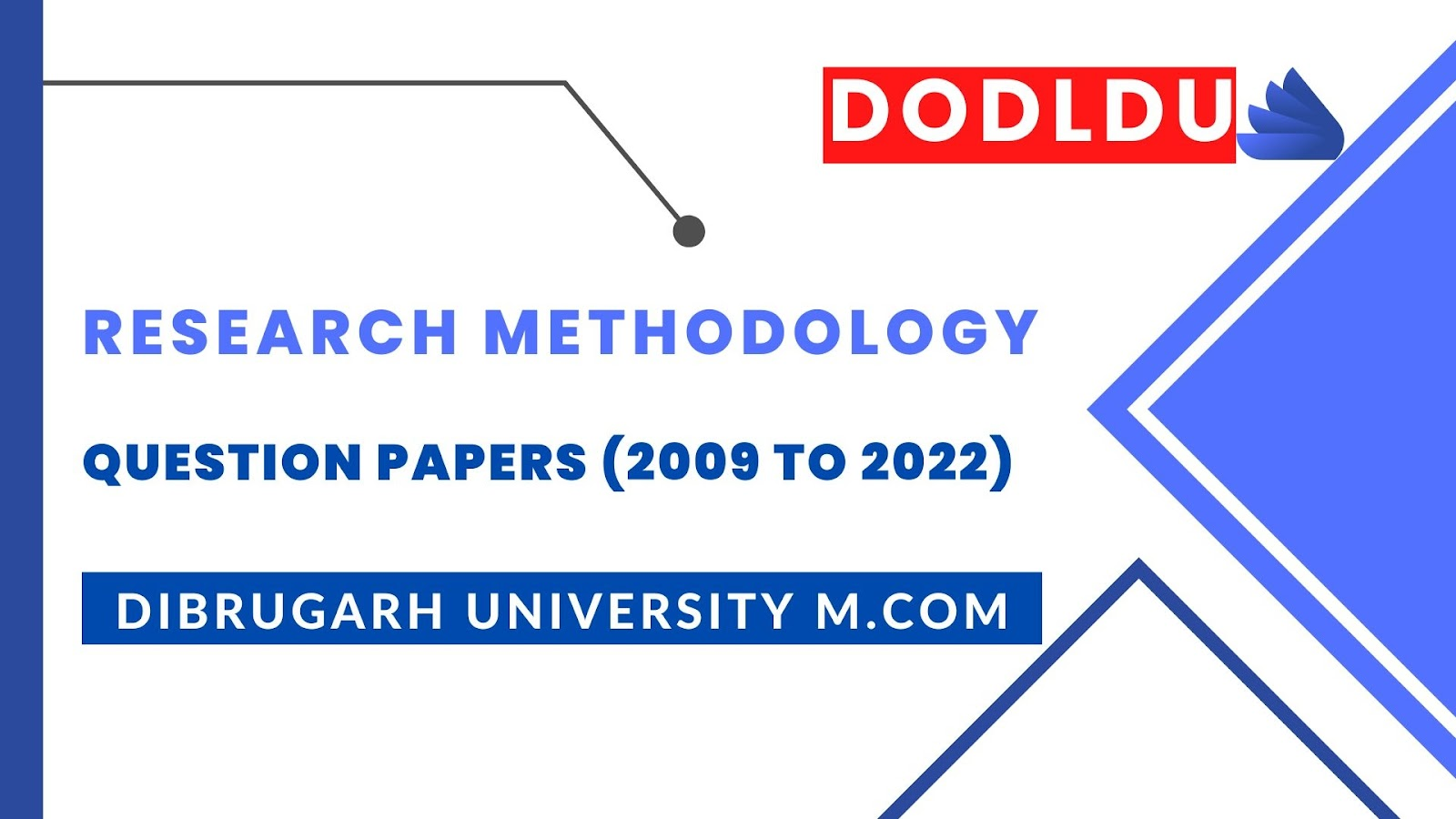# Research Methodology Question Paper 2012 [Dibrugarh University DODLDU M.Com Final Year]## Research Methodology Question Paper 2012 (Nov-Dec)[Dibrugarh University DODLDU M.Com Final Year]

COMMERCE
Paper: 203
(Research Methodology and Statistical Analysis)
Full Marks: 80
Time: 3 hours
The figures in the margin indicate full marks for the questions
1. (a) Define business research. Discuss the need and importance of business research.   6+10=16
Or
(b) What are the methods of collecting primary data? Discuss each of them with examples.    4+12=16

2. (a) Write short notes on:         8x2=16
1)            Measure of Variation.
2)            Measure of Central Tendency.
Or
(b) Define index number and explain briefly the nature of problems involved in the construction of index numbers. How are index numbers useful for businessmen?   3+8+5=16

3.  (a) Discuss the various approaches to probability. Under what conditions is the classical approach not appropriate to employ?           16
Or
(b) Eight coins are tossed at a time 256 times. Number of heads observed at each throw is recorded and the results are given below. Find the expected frequencies. What are the theoretical values of mean and standard deviation? Calculate also the mean and S.D. of the observed frequencies.                     8+2+6=16
 No. of heads at a throw Frequency 0 1 2 3 4 5 6 7 8 2 6 30 52 67 56 32 10 1

4. (a) Define hypothesis. Distinguish between the null and alternative hypothesis. State the null and alternative hypothesis regarding the population means that lead to
1)      Left-tailed test.
2)      Right-tailed test and
3)      Two tailed-test
And briefly explain type I and type II errors.               2+6+4+4=16
Or
(b) In a test given to two groups of students, the marks obtained are as follows:
 1st group: 18 20 36 50 49 36 34 49 41 2nd group: 29 28 26 35 30 44 46 46 47
Examine the significance of difference between the arithmetic mean of the marks secured by the students of the above two groups. (The value of t at 5% level of significance for y=14 is 2.14)     16

5. (a) 4 coins were tossed 160 times and the following results were obtained:                     16
 No. of heads: 0 1 2 3 4 Observed frequency: 17 52 54 31 6
Under the assumption that coins are balanced, find the expected frequencies of getting 0, 1, 2, 3, or 4 heads and test the goodness of fit.            16
Or
(b) Explain the following components of a research report.                    4x4=16
1)            Abstract.
2)            Executive Summary.
3)            Synopsis.
4)            References.
***